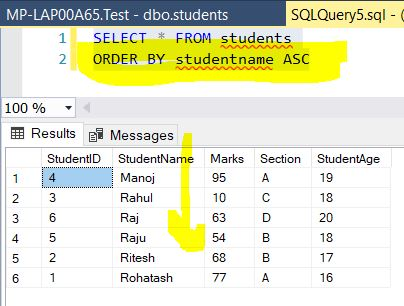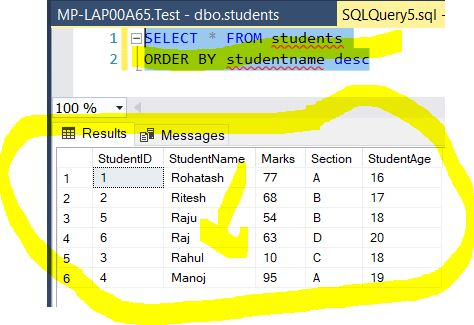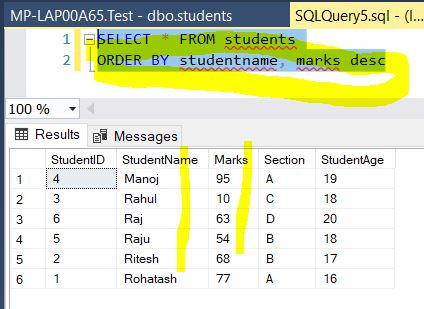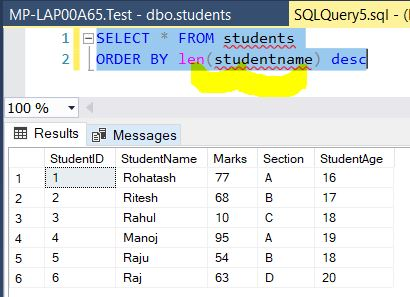﻿ SQL Order By - TutorialsTrend
This Angular tutorial helps you get started with Angular quickly and effectively through many practical examples.
`

# SQL-Order By

In this tutorials, we will learn how to use ORDER BY Clause with multiple columns and expression to sort data in ASC and DESC order.

#### SQL Server ORDER BY Clause

The ORDER BY clause is used to sort the result in ascending or descending order. The ASC sorts the result from the lowest value to the highest value while the DESC sorts the result set from the highest value to the lowest one. The ORDER BY clause sorts the result set in ascending order by default. To sort the records in descending order, use the DESC keyword.

ASC Order

The ASC keyword is used to sort the data returned in ascending order.

The following SQL statement selects all the columns from the students table, sorted by the StudentName column:

Example

``````SELECT * FROM students
ORDER BY studentname ASC``````

OutputDESC Order

The DESC Keyword is used to sort the data returned in descending order.

The following SQL statement selects all the columns from the students table, sorted by the StudentName column:

Example

``````SELECT * FROM students
ORDER BY studentname DESC;``````

Output#### Sort with Multiple Columns

The following statement fetch all columns of the students table. It sorts the customer list by the StudentName first and then by the marks.

Example

``````SELECT * FROM students
ORDER BY studentname, marks desc``````

OutputSort a Result by an Expression

The LEN() function returns the number of characters in a string.

The following statement uses the LEN() function in the ORDER BY clause to retrieve a Student list sorted by the length of the StudentName:

Example

``````SELECT * FROM students
ORDER BY len(studentname) desc``````

OutputNext